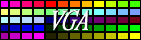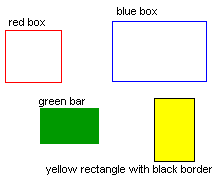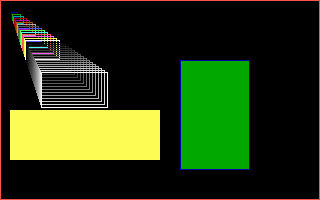## Draw bars and boxesIn my defninition boxes are one pixel wide frames while bars are solid filled boxes. This two can be combined into a third one that has border, so I will call this rectangle.Box

This one is quite simple as we only have to draw two horizontal and two vertical lines. Therefore, I won't use assembly for this trivial task. (Though the asm version could be faster a little bit.)

 ```procedure Box(x,y,width,height,color:integer); begin HorizLine(x,y,width,color); HorizLine(x,y+height-1,width,color); VertLine(x,y,height,color); VertLine(x+width-1,y,height,color); end; ```

I don't think I must explain anything here.

Bar

Well, if you remember how we drew the vertcial line, this one should not be an issue. This time we have to draw vertical lines exactly height times. Thus there are two loops nested here. The first one counts the lines (DX), while the second one (CX) counts the pixels in a line. We're gonna use the STOSB instruction again to show a line, hence it will be fast. Check it out!

 ```procedure Bar(x,y,width,height,color:integer); assembler; asm mov ES,SegA000 { Screen segment } mov ax,320 mul y add ax,x mov bx,ax { Address calculation, store the result in BX } mov al,color.byte cld mov dx,height { height of the bar in the DX } @l0: mov cx,width mov di,bx rep stosb { draw one horizontal line } add bx,320 { address for the next line } dec dx jnz @l0 { loop draws all horiz. lines } end; ```

Rectangle

Well... piece of cake. No assembly.

 ```procedure Rectangle(x,y,width,height,cborder,cfill:integer); begin Bar(x,y,width,height,cfill); Box(x,y,width,height,cborder); end; ```

Test it

At last, this small app draws some objects.

 ```program boxes; uses gfx256; var i : integer; begin graph320x200; box(0,0,320,200,12); for i:=1 to 31 do box(10+i,10+2*i,5+2*i,5+i,i); bar(10,110,150,50,14); rectangle(180,60,70,110,1,2); readln; text80x25; end. ```
This is what we see